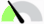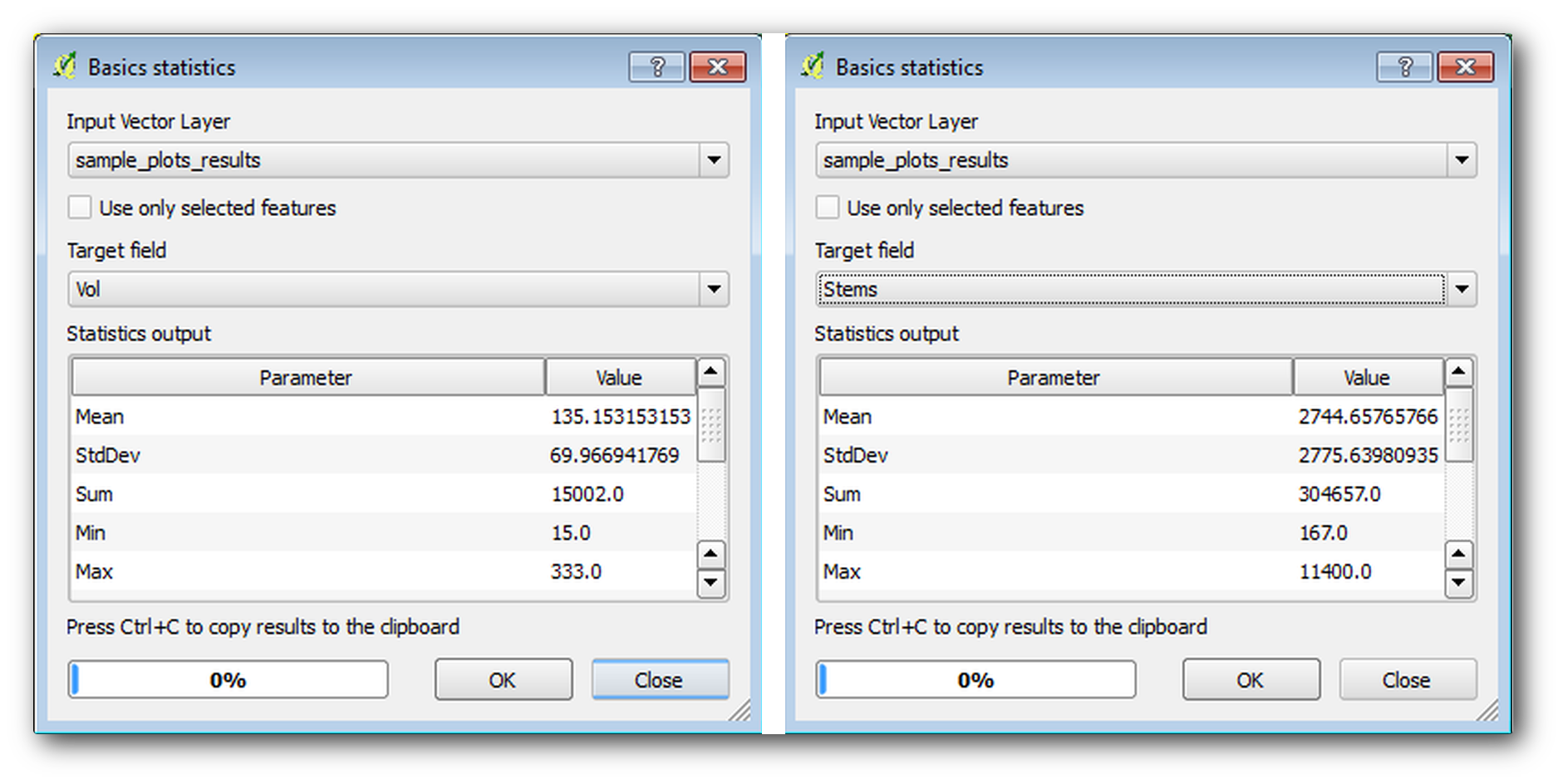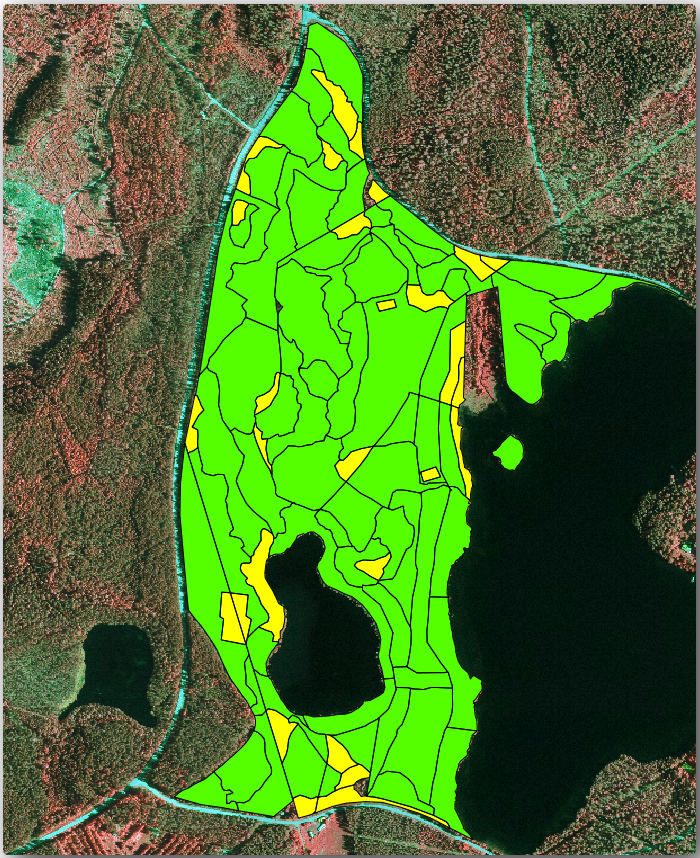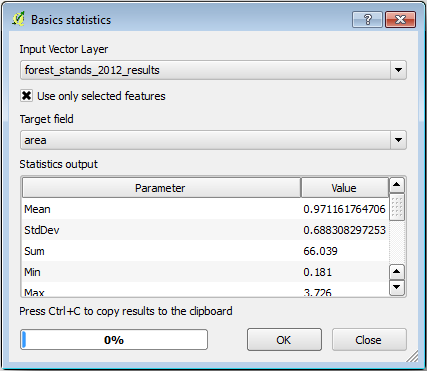# 14.7. Lesson: Calculating the Forest Parameters¶

Estimating the parameters of the forest is the goal of the forest inventory. Continuing the example from previous lesson, you will use the inventory information gathered in the field to calculate the forest parameters, for the whole forest first, and then for the stands you digitized before.

The goal for this lesson: Calculate forest parameters at general and stand level.

## 14.7.1.Follow Along: Adding the Inventory Results¶

The field teams visited the forest and with the help of the information you provided, gathered information about the forest at every sample plot.

Most often the information will be collected into paper forms in the field, then typed to a spreadsheet. The sample plots information has been condensed into a `.csv` file that can be easily open in QGIS.

Continue with the QGIS project from the lesson about designing the inventory, you probably named it `forest_inventory.qgs`.

1. Go to Layer ► Add Delimited Text Layer....

2. Browse to the file `systematic_inventory_results.csv` located in `exercise_data/forestry/results/`.

3. Make sure that the Point coordinates option is checked.

4. Set the fields for the coordinates to the X and Y fields.

5. Click OK.

6. When prompted, select ETRS89 / ETRS-TM35FIN as the CRS.

7. Open the new layer's Attribute table and have a look at the data.

You can read the type of data that is contained in the sample plots measurements in the text file `legend_2012_inventorydata.txt` located in the `exercise_data/forestry/results/` folder.

The `systematic_inventory_results` layer you just added is actually just a virtual representation of the text information in the `.csv` file. Before you continue, convert the inventory results to a real spatial dataset:

1. Right click on the `systematic_inventory_results` layer.

2. Browse to `exercise_data/forestry/results/` folder.

3. Name the file `sample_plots_results.shp`.

4. Check Add saved file to map.

5. Remove the `systematic_inventory_results` layer from your project.

## 14.7.2.Follow Along: Whole Forest Parameters Estimation¶

You can calculate the averages for this whole forest area from the inventory results for the some interesting parameters, like the volume and the number of stems per hectare. Since the systematic sample plots represent equal areas, you can directly calculate the averages of the volumes and number of stems per hectare from the `sample_plots_results` layer.

You can calculate the average of a field in a vector layer using the Basic statistics tool:

1. Open Vector ► Analysis Tools ► Basic statistics for Fields.

2. Select `sample_plots_results` as the Input Vector Layer.

3. Select `Vol` as Target field.

4. Click OK.

The average volume in the forest is `135.2 m3/ha`.

You can calculate the average for the number of stems in the same way, `2745 stems/ha`.## 14.7.3.Follow Along: Estimating Stand Parameters¶

You can make use of those same systematic sample plots to calculate estimates for the different forest stands you digitized previously. Some of the forest stands did not get any sample plot and for those you will not get information. You could have planned some extra sample plots when you planned the systematic inventory, so that the field teams would have measured a few extra sample plots for this purpose. Or you could send a field team later to get estimates of the missing forest stands to complete the stand inventory. Nevertheless, you will get information for a good number of stands just using the planned plots.

What you need is to get the averages of the sample plots that are falling within each of the forest stands. When you want to combine information based on their relative locations, you perform a spatial join:

1. Open the Vector ► Data Management ► Join attributes by location tool.

2. Set `forest_stands_2012` as the Target vector layer. The layer you want the results for.

3. Set `sample_plots_results` as the Join vector layer. The layer you want to calculate estimates from.

4. Check Take summary of intersecting features.

5. Check to calculate only the Mean.

6. Name the result as `forest_stands_2012_results.shp` and save it in the `exercise_data/forestry/results/` folder.

7. Finally select Keep all records..., so you can check later what stands did not get information.

8. Click OK.

10. Close the Join attributes by location tool.

Open the Attribute table for forest_stands_2012_results and review the results you got. Note that a number of forest stands have `NULL` as the value for the calculations, those are the ones having no sample plots. Select them all and view them in the map, they are some of the smaller stands:Lets calculate now the same averages for the whole forest as you did before, only this time you will use the averages you got for the stands as the bases for the calculation. Remember that in the previous situation, each sample plot represented a theoretical stand of `80x80 m`. Now you have to consider the area of each of the stands individually instead. That way, again, the average values of the parameters that are in, for example, m3/ha for the volumes are converted to total volumes for the stands.

You need to first calculate the areas for the stands and then calculate total volumes and stem numbers for each of them:

1. In the Attribute table enable editing.

2. Open the Field calculator.

3. Create a new field called `area`.

4. Set the Output field type to `Decimal number (real)`.

5. Set the Precision to `2`.

6. In the Expression box, write `\$area / 10000`. This will calculate the area of the forest stands in ha.

7. Click OK.

Now calculate a field with the total volumes and number of stems estimated for every stand:

1. Name the fields `s_vol` and `s_stem`.

2. The fields can be integer numbers or you can use real numbers also.

3. Use the expressions `"area"  *  "MEANVol"` and `"area"  *  "MEANStems"` for total volumes and total stems respectively.

4. Save the edits when you are finished.

5. Disable editing.

In the previous situation, the areas represented by every sample plot were the same, so it was enough to calculate the average of the sample plots. Now to calculate the estimates, you need to divide the sum of the stands volumes or number of stems by the sum of the areas of the stands containing information.

1. In the Attribute table for the `forest_stands_2012_results` layer, select all the stands containing information.

2. Open Vector ► Analysis Tools ► Basic statistics for fields.

3. Select the `forest_stands_2012_results` as the Input layer.

4. Select `area` as Field to calculate statistics on.

5. Check the Selected features only

6. Click OK.As you can see, the total sum of the stands' areas is `66.04 ha`. Note that the area of the missing forest stands is only about `7 ha`.

In the same way, you can calculate that the total volume for these stands is `8908 m3/ha` and the total number of stems is `179594 stems`.

Using the information from the forest stands, instead of directly using that from the sample plots, gives the following average estimates:

• `184.9 m3/ha` and

• `2719 stems/ha`.

Save your QGIS project, `forest_inventory.qgs`.

## 14.7.4. In Conclusion¶

You managed to calculate forest estimates for the whole forest using the information from your systematic sample plots, first without considering the forest characteristics and also using the interpretation of the aerial image into forest stands. And you also got some valuable information about the particular stands, which could be used to plan the management of the forest in the coming years.

## 14.7.5. What's Next?¶

In the following lesson, you will first create a hillshade background from a LiDAR dataset which you will use to prepare a map presentation with the forest results you just calculated.